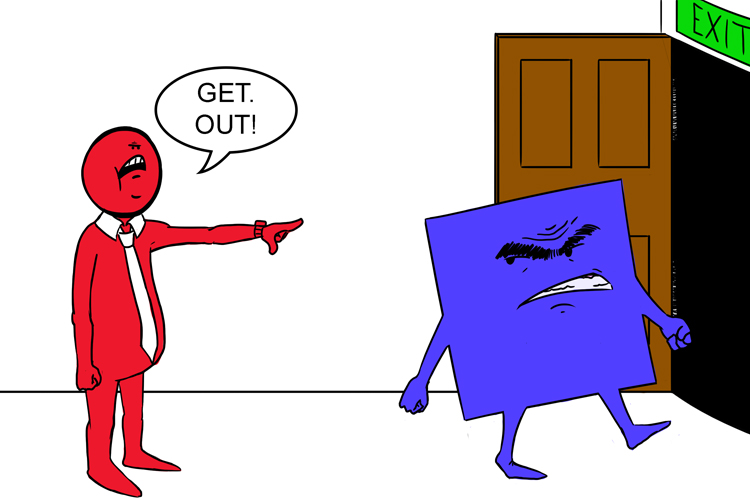# Complete the square – Getting rid of the square (easily)

## (the first of three ways to solve (find the roots) of quadratics)Completely get rid of the square

When it’s easy we complete the square as follows:

Examples

x^2=9                    Solve    x=sqrt9          x=+-3

x^2=5                    Solve    x=sqrt5

(x-7)^2=3        Solve    x=7+-sqrt3

See how we completely got rid of the square

Get any quadratic in the form
(x-7)^2
then we can find the values of x.